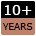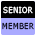Welcome to WebmasterWorld Guest from 54.91.71.108

Forum Moderators: coopster & jatar k

Message Too Old, No Replies

Script Help

bold php

airpirate

9:47 pm on Sep 9, 2008 (gmt 0)

New Userjoined:Sept 9, 2008
posts: 8

I am a beginner at PHP, self taught, no formal classes. I am putting together a task page for myself with the following code. I would like to figure out a way that to have the task highlighted or bold-ed when it is today's date or past today's date. Any help would be appreciated.

<?php

@\$today = date('d/m/y');
@\$pfw_host = "localhost";
@\$pfw_user = "user";
@\$pfw_pw = "pass";
@\$pfw_db = "db";
{
die('Could not connect: ' . mysql_error());
}

if (!\$pfw_db_selected)
{
die ('Can not use \$pfw_db : ' . mysql_error());
}

\$query=mysql_query("select id, task, due_date From tasks where completed is Null and to_do ='X' order by due_date;") or die ("invalid query");

print'
<center>
<table align=center border=0 cellpadding=0 cellspacing=2 width=600>
<tr align=center>
<td width=100 align=center><b>Complete</b></td>
<td width=100 align=center><b>Due Date</b></td>
<tr><td colspan="10" bgcolor="black" height="1"></td></tr>
';

while(\$row=mysql_fetch_array(\$query)){
if(\$color == 1)
{
print '<tr bgcolor=#999966>
<FORM METHOD="post" ACTION="complete.php">
<td align=center><input type="submit" name="button" value= "Mark Complete" /></td>
<td align=center> ' . \$row['task'] . ' </td>
<td align=center> ' . \$row['due_date'] . ' </td>
<input name="id" type="hidden" value="' . \$row['id'] . '">
</form>
</tr>';
\$color=0;
}
else
{
print '<tr>
<FORM METHOD="post" ACTION="complete.php">
<td align=center><input type="submit" name="button" value= "Mark Complete" /></td>
<td align=center> ' . \$row['task'] . ' </td>
<td align=center> ' . \$row['due_date'] . ' </td>
<input name="id" type="hidden" value="' . \$row['id'] . '">
</form>
</tr>';
\$color=1;

}
}

?>

cameraman

10:16 pm on Sept 9, 2008 (gmt 0)

Senior Memberjoined:Jan 16, 2007
posts:914

Welcome to Webmaster World, airpirate.

First off, how about using CSS to simplify a bit. In the head of the document,
<style type="text/css">
.alt { background-color: #999966; }
.hi { background-color: #FFFFF0; }
</style>

\$color = 1;
while(\$row=mysql_fetch_array(\$query)){
\$class = \$color ? '' : 'alt';
\$color = 1 - \$color;

print '<tr class="' . \$class . '">

<FORM METHOD="post" ACTION="complete.php">
<td align=center><input type="submit" name="button" value= "Mark Complete" /></td>
<td align=center> ' . \$row['task'] . ' </td>
<td align=center> ' . \$row['due_date'] . ' </td>
<input name="id" type="hidden" value="' . \$row['id'] . '">
</form>
</tr>';
}

\$color = 1;
while(\$row=mysql_fetch_array(\$query)){
\$class = \$color ? '' : 'alt';
\$color = 1 - \$color;
\$today = strtotime("11:59:59pm");
\$class = 'hi';
print '<tr class="' . \$class . '">

<FORM METHOD="post" ACTION="complete.php">
<td align=center><input type="submit" name="button" value= "Mark Complete" /></td>
<td align=center> ' . \$row['task'] . ' </td>
<td align=center> ' . \$row['due_date'] . ' </td>
<input name="id" type="hidden" value="' . \$row['id'] . '">
</form>
</tr>';
}

This bit:
\$class = \$color ? '' : 'alt';
makes use of a ternary operator that evaluates the expression to the left of the question mark. If the expression is true, the result will be the stuff between question mark and colon, and if false, then the result will be the stuff to the right of the colon.

As a side note, you can do this sort of thing:
<?php
\$a = 7;
\$b = 3;
?>
<p>The first is <?php echo \$a; ?> and the second is <?php echo \$b; ?>.</p>
<?php
\$c = \$a + \$b;
?>
<p>And the sum is <?php echo \$c; ?>.</p>

In other words, you can switch back and forth between html and php. There's nothing wrong with how you've done it, but it would be easy to get a quote in the wrong place and a pain to track down where it's supposed to go.

MattAU

10:26 pm on Sept 9, 2008 (gmt 0)

Junior Memberjoined:June 6, 2005
posts:109

Using strtotime() is a great way to compare dates, because it makes them into an integer timestamp.

You could use something like this:

\$today_stamp = strtotime(\$today);
if(strtotime(\$row['due_date']) >= \$today_stamp)
//make bold
}

I noticed a couple of other little issues with your code:

\$variables need to be in "double quotes" to be parsed. So ['Can not use \$pfw_db : '] will actual output [Can not use \$pfw_db : ] not [Can not use 01/01/08: ]. The square brackets are not part of the output, I just used them for quoting so it didn't get too confusing using quotes to quote quotes about quotes... :)

The @ symbol is used for supressing runtime errors, but it won't do anything when you're assigned a plain text string eg. \$pfw_host = "localhost"; If there's an error in that code it'll be a parse error so the script won't run anyway. Because of this @ has no use in those first for lines.

Hope this helps :)

airpirate

6:43 pm on Sept 10, 2008 (gmt 0)

New Userjoined:Sept 9, 2008
posts: 8

Thanks guys! That helps me a lot.